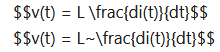# Complex Fractions in LaTeX

• LaTeX
• topsquark
Of course it didn’t, spaces are mostly irrelevant to math mode (for good reason).For integrals, using the physics package might be helpful to some extent. One may use \dd{} from that package, which manages the spacing automatically, and also makes the d upright.f

#### topsquark

Gold Member
MHB
TL;DR Summary
Exploring different ways to represent a complex fraction using LaTeX
I know of two reasonable ways to represent a complex fraction:
\dfrac{ \left ( \dfrac{a}{b} \right ) }{ \left ( \dfrac{c}{d} \right ) }
##\dfrac{ \left ( \dfrac{a}{b} \right ) }{ \left ( \dfrac{c}{d} \right ) }##

and
\dfrac{ ^a / _b }{ ^c / _d }
##\dfrac{ ^a / _b }{ ^c / _d }##

What I am wondering is if there is a way to write
##\dfrac{ \dfrac{a}{b} }{ \dfrac{c}{d} }##
by making a longer middle division line?

Thanks!

-Dan

Hey Dan,

One way to make the middle line longer, is to add latex spaces to numerator or denominator.
For instance:
\dfrac{\;\dfrac ab\;}{\dfrac cd}
##\dfrac{\;\dfrac ab\;}{\dfrac cd}##

••topsquark and berkeman
add latex spaces to numerator or denominator
Sometimes I have to add explicit "~" characters to force spaces in LaTeX, but other times just " " spaces seem to work. I haven't figured out why yet...

•topsquark
Sometimes I have to add explicit "~" characters to force spaces in LaTeX, but other times just " " spaces seem to work. I haven't figured out why yet...
Can you give some examples?

Sometimes I have to add explicit "~" characters to force spaces in LaTeX, but other times just " " spaces seem to work. I haven't figured out why yet...
Regular spaces are generally used as separator. For instance in \dfrac ab the space is not an actual space, but instead it's a separator. That separator only "becomes" a space, if that is needed to separate both sides. In that case multiple spaces are collapsed to a single space.
If we actually "want" a space where that is not already natural, we have to use one of the latex spaces like:
\! \, \: \; \quad \qquad, which are increasingly wide spaces. The first one is a negative space. The "~" is special in that it creates a non-breaking space, so that it won't "wrap".

••Orodruin, berkeman and topsquark
Can you give some examples?
Just a quick example:
$$v(t) = L \frac{di(t)}{dt}$$
$$v(t) = L~\frac{di(t)}{dt}$$
from:The classical example is an integral: $$\int f(x,y) dx dy$$
Often \, is inserted (despite already having spaces) before the infinitesimals to get a more natural formula:$$\int f(x,y)\,dx\,dy$$
A "~" also works of course.

•pbuk and topsquark
Just a quick example:
$$v(t) = L \frac{di(t)}{dt}$$
$$v(t) = L~\frac{di(t)}{dt}$$
from:
View attachment 313827
I meant examples of where you would expect a space but there was none.

A "~" also works of course.
Compare the following:
$$\int f(x,y)~dx~dy \qquad \int f(x,y)\,dx\,dy$$
The non-breaking space ~ is a regular sized space (it is equivalent in size to the regular \ ) as compared to \, which is 3 mu. The latter is typically what you want. The non-breaking space ~ should be used when you have a space where you do not wish to create a (line or page) break. In an equation it is essentially equivalent to the regular space "\ " (which has its own uses in text as well, such as not creating a too large space after an abbreviation, i.e., LaTeX will interpret "cf. last chapter" as there being an end of sentence after cf. whereas "cf.\ last chapter" will not).

•I like Serena and topsquark
I meant examples of where you would expect a space but there was none.
In the first LaTeX line, I put a " " space after the L, but it did not render. It took the explicit "~"space after the L to get it to render.

In the first LaTeX line, I put a " " space after the L, but it did not render. It took the explicit "~"space after the L to get it to render.
Of course it didn’t, spaces are mostly irrelevant to math mode (for good reason).

For integrals, using the physics package might be helpful to some extent. One may use \dd{} from that package, which manages the spacing automatically, and also makes the d upright.
$$\require{physics} \int f(x,y) \dd{x} \dd{y}$$

•topsquark